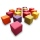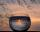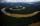# Geometry - math word problems

#### Number of problems found: 1318

• Two wallsCalculate the surface area of a cube in m2 if you know that the area of its two walls is 72 dm2.
• RainHow many mm of water rained the roof space 75 m2 if the empty barrel with a radius of 8 dm and height 1.2 m filled to 75% its capacity? :-)
• The potThe pot is a cylinder with a volume of V = 7l and an inner diameter of d = 20cm. Find its depth.
• DiameterWhat is the inside diameter of the cylinder container and if half a liter of water reaches a height 15 cm?
• PotCalculate the height of 3 liter pot with shape cylinder with a diameter of 10 cm.
• Liters in cylinderDetermine the height at which level 24 liters of water in a cylindrical container having a bottom diameter 36 cm.
• TroughHow many liters of water per second can go via trough, which has a cross section of semicircle with radius 2.5 m and speed of water is 147 cm per second?
• Gold wireFrom one gram of gold was pulled wire 2.1 km length. What is it diameter if density of Au is ρ=19.5 g/cm3?
• Two ribbonsThe total length of the two ribbons is 13 meters. If one ribbon is 7 and 5/8 meters long, what is the length of the other ribbon?
• Cone 15The radius of the base of a right circular cone is 14 inches and it's height 18 inches. What is the slant height?
• Inscribed sphereHow many percent of the cube volume takes the sphere inscribed into it?
• Water flowHow much water flow in pipe with a diameter of 16 cm in 1 hour if the water velocity is 2.5 m/s?
• CubeThe sum of lengths of cube edges is 57 cm. What is its surface and volume?
• Cube in sphereThe sphere is inscribed cube with edge 8 cm. Find the radius of the sphere.
• Tank and waterCylindrical tank were poured with 3.5 liters of water. If tank base diameter is 3 dm, how height is water level in?
• Hard cone problemThe surface of the cone is 200cm², its height is 7 centimeters. Calculate the volume of this cone.
• Cube edgesIf the edge length of the cube increases by 50%, how does the volume of this cube increase?
• ConvaHow many liters of water fit into the shape of a cylinder with a bottom diameter 20 cm and a height 45 cm?
• RhombusABCD is a rhombus, ABD is an equilateral triangle and AC is equal to 4. Find the area of the rhombus.
• Cuboid aquariumCuboid 25 times 30 cm. How long is third side if cuboid contains 30 liters of water?

Do you have an interesting mathematical word problem that you can't solve it? Submit a math problem, and we can try to solve it.

We will send a solution to your e-mail address. Solved examples are also published here. Please enter the e-mail correctly and check whether you don't have a full mailbox.

Please do not submit problems from current active competitions such as Mathematical Olympiad, correspondence seminars etc...